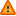FAQ/Mauchly - CBU statistics WikiYou should trust both wikis because the password could be read by the particular administrators.

Clear message
location: FAQ / Mauchly

# What is the formula for Mauchly's W used for testing sphericity in univariate repeated measures anova?

Mauchley's test of sphericity is used to test the assumption in univariate repeated measures that the covariance matrix of the repeated measures has a particular form (see the Mixed Anovas talk in the Graduate Statistics Talk Series.) The violation of sphericity occurs when it is not the case that the variances of the differences between all combinations of the groups are equal. A special, more extreme, form of sphericity is compound symmetry which is a more stringent than necessary assumption that the variances of the levels of repeated measures factors are equal to a value possibly different to that of the covariances which are also assumed equal.

After the W is computed, the “chi-square” (χ2) distribution may be used to assess whether the data show a statistically significant difference from sphericity.

This measure reports if there is a correlation between pairs of repeated measures. If the observed statistic for Mauchly’s test is statistically significant and the underlying distributions are normal, then the assumption of sphericity is rejected and an adjustment such as Greenhouse-Geisser or Huynh-Feldt will be necessary. It may be useful to switch to the multivariate form of repeated measures ANOVA in which sphericity is not assumed.

The test statistic, W, is compared to a chi-square distribution to assess the adequacy of the sphericity assumption.

The formula for W for a k x k covariance matrix, S, with determinant det(S), trace (or sum of the variances of S), tr(S), equals:

$$W = det(S)( \frac{k+1}{tr(S)})^text{k+1}$$

It should be mentioned that although Mauchly’s test is a popular tool, it has been criticized for its inaccuracy when multivariate normality cannot be assured (Keselman, Rogan, Mendoza, & Breen, 1980; Rogan, Keselman, & Mendoza, 1979). Thus, prudent researchers need to be careful about mechanical use of this test. It makes sense to check the underlying normality of the distributions of data (and the transformed variables tracking the differences between each pair of repeated measures) before relying on the Mauchly test.

References

Maxwell S. E., Delaney H. D. (2004). Designing Experiments and Analyzing Data: A Model Comparison Perspective, 2nd Edn. Mahwah, NJ: Erlbaum

Nimon K., Williams C. (2009). Performance improvement through repeated measures: a primer for educators considering univariate and multivariate design. Res. High. Educ. J. 2, 117–136 (pre-print attached)